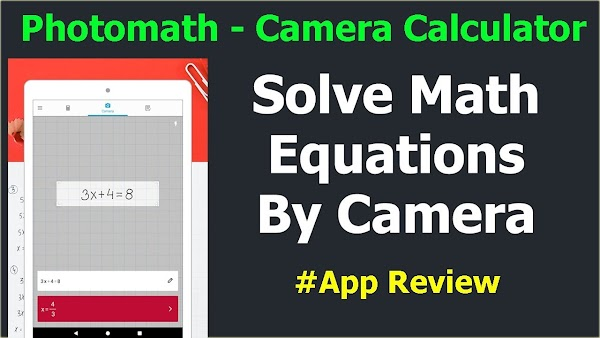## Featured Post

### FIFA 21 Mobile Official by Tencent Best Graphics Download

Play Every Moment! “FIFA Mobile” officially opened! Play Every Moment “FIFA Mobile” FIFA Mobile is not only fun to build and grow your own t...## Math Caluclator - Solve Math Problem by Photo

### Math Caluclator - Solve Math Problem by Photoadmin
Saturday, October 26, 2019
Math Calculator can help you solve math homework assignments, check answers, and solve multiple questions with just one tap. Simply use the camera as a scanner and scan your math problems, Math Calculator will show you the solutions instantly. Aside from the basic and scientific calculators, there are also other very helpful features, such as equation solver, calorie scanner, and unit converter. Math Calculator is completely FREE to use, and is a great help for work and study.

HOW IT WORKS?
Scan printed or handwritten math problems using your phone or tablet’s camera, and Calculator All-In-One will show you the solutions right away. You can scan multiple lines of problems and multi-variable equations as well, and they will all be solved instantly. We also have a built-in equation solver that lets you type in the equations to solve. For quadratic equations, all solutions will be displayed. We solve your problems 100%.

KEY FEATURES
Scan to Solve: Use camera to scan math problems for solutions. No need to type, solve math problems with just one tap.
Equation Solver: Solve quadratic equations and multi-variable equations. All solutions will be displayed if there are more than one solution to the equation.
Answer Checker: Scan your homework after you do it to see if there are any wrong answers.
Basic & Scientific Calculator: We have everything a calculator has to help you solve basic and advanced math problems.
Calculation History: Check your history anytime incase you need to back track your calculations.
Calorie & Object Scanner: Scan food for its calorie count, and scan anything you don’t know the name to search for more info.
Unit Converter: All units available for you to choose and convert.

Math Caluclator - Solve Math Problem by Photo
4/ 5
Oleh

Name

Email *

Message *